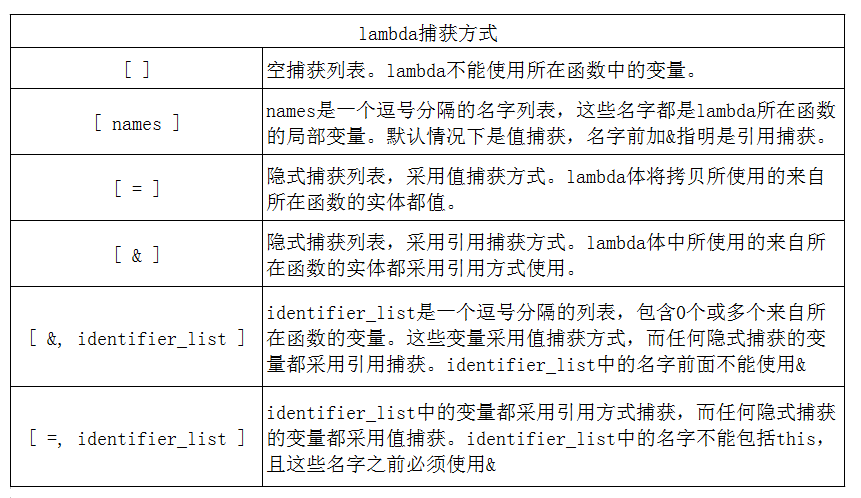# 概述

C++ 11中引入了新的lamdba表达式，使用也很简单，我最喜欢的是不用给函数取名称，每次给函数取名称都感觉自己读书太少~

# 1、lambda表达式

lambda表达式可以理解为一个匿名的内联函数。和函数一样，lambda表达式具有一个返回类型、一个参数列表和一个函数体。与函数不一样的是lambda必须使用尾置返回类型。一个lambda表达式表示一个可调用的代码单元。

capture list：表示捕获列表，是一个lambda所在函数中定义的局部变量列表
parameter list：表示参数列表
return type：返回类型
function body：函数体

auto f = [ ] {return 42;};
cout << f() << endl;


lambda的调用方式与普通函数的调用方式相同。

1. lambda表达式不能有默认参数。因此，一个lambda表达式调用的实参数目永远与形参数目相等
2. 所有参数必须有参数名
3. 不支持可变参数

# 2、捕获列表

1. lambda只有在其捕获列表中捕获一个它所在函数中的局部变量，才能在函数体中使用该变量
2. 捕获列表只用于非静态局部变量，lambda可以直接使用静态局部变量和在它所在函数之外声明的名字

void func()
{
static int i = 10;
int  j = 20;
auto f1 = [ ] () { return j; };		//编译出错，没有进行捕获，函数体内不能使用
auto f2 = [ ] () { return i; };		//静态变量无需捕获
auto f3 = [j] () { return j; };		//进行了捕获，函数体内可以使用了
}；## 2.1、值捕获

void func()
{
size_t v1 = 42;
auto f = [ v1 ]  { return v1; };	//使用了值捕获，将v1拷贝到名为f的可调用对象
v1 = 0;
auto j = f();	//j为42，f保存了我们创建它是v1的拷贝
}


## 2.2、引用捕获

void func()
{
size_t v1 = 42;
auto f = [ &v1 ]  { return v1; };	//引用捕获，将v1拷贝到名为f的可调用对象
v1 = 0;
auto j = f();	//j为42，f保存了我们创建它是v1的拷贝
}


## 2.3、隐式捕获

int main()
{
int a = 123;
auto f = [ = ]  { cout << a << endl; };		//值捕获
f(); 	// 输出：123

auto f1 = [ & ] { cout << a++ << endl; }; 		//引用捕获
f1();	//输出：123（采用了后++）

cout << a << endl; 		//输出 124
}


## 2.4、混合方式捕获

lambda还支持混合方式捕获，即同时使用显示捕获和隐式捕获

void func()
{
int i = 10;
int  j = 20;
auto f1 = [ =, &i] () { return j + i; };		//正确，默认值捕获，显示是引用捕获
auto f2 = [ =, i] () { return i + j; };		//编译出错，默认值捕获，显示值捕获，冲突了
auto f3 = [ &, &i] () { return i +j; };		//编译出错，默认引用捕获，显示引用捕获，冲突了
}；


## 2.5、修改值捕获的值

int main()
{
int a = 123;
auto f = [a]()mutable { cout << ++a; }; // 不会报错

cout << a << endl; 	// 输出：123
f(); 					// 输出：124
}


# 3、返回类型

1. 如果只包含单一的return语句，那么根据return 的类型确定返回类型。
2. 如果除了return 还有别的语句，那么返回void。

# 4、lambda表达式的本质

## 4.1、采用值捕获

int func()
{
int a =10;
int b = 20;
auto addfun = [=] (const int c ) -> int { return a+c; };

int c = addfun(b);
cout << c << endl;
};


class Myclass
{
public:
Myclass( int a ) : m_a(a){};	//该形参对应捕获的变量

//该调用运算符的返回类型、形参和函数体都与lambda一致
int operator()(const int c) const
{
return a + c;
}

private:
int m_a;		//该数据对应通过值捕获的变量
};


lambda表达式产生的类不含有默认构造函数、赋值运算符及默认析构函数。因为不含默认构造函数，因此要想使用这个类必须提供一个实参。

## 4.2、采用引用捕获

《C++ primer 第五版》
https://www.cnblogs.com/lustar/p/7531605.html10-29
12-142万+
11-041万+
06-037658
03-262014
07-306579
04-061万+
07-191002
02-223507
09-207996
07-033万+
09-03470
04-08868
09-2077
06-17227
©️2020 CSDN 皮肤主题: 游动-白 设计师:白松林